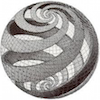# The Developer’s Cry

Yet another blog by a hobbyist programmer

# Round Balls and Flat DisksI used to call `gluSphere()` to render simple planets in 3D space programs. For reasons beyond me, this function has been deprecated. Apparently, all GLU functions have been scrapped in the newer OpenGL standard. I’ve been told by an expert that OpenGL maintains backward compatibility by using version numbering, meaning that you should still be able to use old OpenGL 2, as long as you don’t try to mix it with OpenGL 4 functions. GLU and GLUT are not really part of the OpenGL standard so that’s where the story ends, I suppose. The problem is, I found myself being unable to compile older programs anymore after upgrading the operating system—totally unexpected, an unpleasant surprise. Searching for an answer, I found StackOverflow. The answer: “Do the math.”

I had to scratch my head a little there. I have to admit, it’s been a while since I ‘did the math’ on anything, really. A visit to MathWorld turned up some nice formulas. Now, how to put that into code.

## Implementing gluSphere()

As you can see in wireframe mode, the gluSphere is built up from quads. Clearly, triangle strips speed up the process. It should be possible to use a single triangle strip, but I didn’t bother trying to figure that one out. So I went for stacked triangle strips that wrap around the sphere. This method uses slices and stacks (similar to lines of longitude and latitude) just like `gluSphere()` does.

Anyway, the math. We need to calculate all the vertices for the triangle strips that make up the sphere. A sphere is like rotating a vector 360 degrees around in a horizontal plane (left, right), while rotating a vector 180 degrees up and down in a vertical plane. We’ll call the first angle alpha and the second beta. The size of the sphere is determined by radius `r`. Any point (x, y, z) on the sphere is given by:

``````x = r * cos alpha * sin beta
y = r * cos beta
z = r * sin alpha * sin beta
``````

One caveat is that the angles should be given in radians rather than degrees for the C math library, so use `M_PI`. Another is the OpenGL coordinate system; you may have to work with -z, or like in my case, I had to exchange the Y and Z-axis to get the desired result.

Knowing this, calculating all vertices is easy. Setup a double loop that calculates all coordinates, taking into a account the number of stacks and slices that you wish to have.

``````for (beta = 0.0; beta <= M_PI; beta += M_PI/num_stacks) {
for (alpha = 0.0; alpha <= 2.0*M_PI; alpha += 2.0*M_PI/num_slices) {
calculate x, y, z
store in vertex array
}
}
``````

## Calculating Sphere Normals

The get good lighting on the sphere, we need to know the normal vectors. The normal at each vertex is given by `normalize(x,y,z)`. That’s easy.

## Calculating Sphere Texture Coordinates

Having planets without texture is not fun. Texture coordinates run from 0.0 to 1.0.

``````x = alpha / (2.0 * M_PI)
y = (M_PI - beta) / M_PI
``````

## Implementing gluDisk()

For the rings of Saturn, it’s easy to use a gluDisk. `gluDisk()` renders a flat disk, with an inner hole in the center. In math terms, the disk uses a circle formula, and there are two radii (one for the outer circle, one for the inner hole). So a gluDisk is really nothing but a single triangle strip that loops around, and its vertices are determined by two circles with radius `r1` and `r2`.
The vertices are given by:

``````// inner vertex
ax = r1 * cos alpha
ay = r1 * sin alpha
az = 0.0

// outer vertex
bx = r2 * cos alpha
by = r2 * sin alpha
bz = 0.0
``````

## Calculating Disk Normals

The disk is really a 2D object in 3D space. The normals point to +Z, they are all defined as (0, 0, 1).

## Calculating Disk Texture Coordinates

Texturing a disk is not at all like texturing a sphere. When you put a texture on a gluDisk, it’s like sticking a label onto a DVD. You can take a rectangular image and put it onto the circular disk without distorting the image or wrapping it around the object. The texture is mapped to the bounding rectangle of the disk, which is two times the outer radius.

``````x = ax / (2.0 * r2) + 0.5
y = ay / (2.0 * r2) + 0.5
``````

Do the same for `bx` and `by`. Note the plus one half, you want to be in the center of the texel or else OpenGL may show artifacts.

## Implementing gluCylinder()

I did not implement `gluCylinder()`, but with the information given for `gluDisk()` you should be able to pull it off. It’s just two circles and an added Z-axis.

## Roundup

So, that’s it. A bit of simple math, but still a sizable amount of work to get spheres going again in OpenGL. It’s something that any serious 3D programmer should be able to do, but I still feel a bit bummed that they just deprecated those really useful functions.

On a final note I’d like to mention that there is another way of creating spheres, one that presumably looks better. Imagine an icosahedron, and subdividing each face into more triangles to enhance resolution. You can subdivide as many times as you like, and at a certain point it will be a good approximation of a sphere. This method is described in the Red Book.